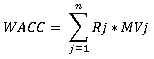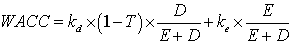# The Weighted Average Cost of Capital and its Computation

WACC (Weighted average cost of capital) is an indicator, which is primarily being used for the estimation of investment into various stocks, projects and discounting the expecting investments. In other words, it is the total cost of capital computed as the total of return on equity and return on debt, weighted by their share in the capital structure.

WACC helps to estimate the effect from certain decisions. A company can make any investment action if its profitability isn’t lower than current weighted average cost of capital.

Also, WACC measures the alternative cost of investing. It shows the level of profitability that can be achieved by investing in already existing project instead of a new one. WACC can be computed by following formula:where,

• Rj – price of the j-th source of funds;
• MVj – weight of the j-th source of funds.

Classic WACC formula looks as follows:where,

• kd – market rate for the debt capital used by the company;
• T – profit tax rate;
• D – debt capital;
• E -equity;
• ke - required rate of return on equity, %.

Cost of capital shows the investments profitability level, necessary to ensure maximum market value of the company. The weighted average cost of capital indicator integrates the information about the structure elements of formed capital, their individual value and weight in the total amount of capital. It defines the relative level of expenses (reflected as interest payments, dividends, rewards, etc.) for usage of the funds invested in the company operations.

Main difficulty in WACC computation is measuring the price of a capital unit, obtained from the certain source of funds, since this affects the accuracy of calculations. For some sources of funds this price can be precisely estimated (for example, the cost of the bank loan); for others analytic can get only approximate value. However, even approximate measurements of WACC are acceptable to analyze the current situation (they are useful for the comparative analysis of the investment efficiency in the company; also they can be used for the company’s investment policy validation).